Custom SearchSQUARE ROOTS Taking the square root of a number with the slide rule is the inverse process of squaring a number. We find the number on the A scale, set the hairline of the indicator over it, and read the square root on the D scale under the hairline. Positioning Numbers on the A Scale Since there are two parts of the A scale exactly alike and the digit sequence could be found on either part, a question arises as to which section to use. Generally, we think of the left half of the rule as being numbered from 1 to 10 and the right half as being numbered from 10 to 100. The numbering continues- left half 100 to 1,000, right half 1,000 to 10,000, and so forth. A simple process provides a check of the location of the number from which the root is to be taken. For whole or mixed numbers, begin at the decimal point of the number and mark off the digits to the left (including end zeros) in groups of two. This is illustrated in the following two examples: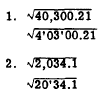Look at the left-hand group. If it is a l-digit number, use the left half of the A scale. If it is a a-digit number, use the right half of the A scale. The number in example 1 is thus located in the left half of the A scale and the number in example 2 is located in the right half. Numbers Less Than One For positive numbers less than one, begin at the decimal point and mark off groups of two to the right. This is illustrated as follows: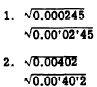Looking from left to right, locate the first group that contains a digit other than zero. If the first figure in this group it3 zero, locate the number in the left half of the A scale. If the first figure is other than zero, locate the number in the right half of the A scale. Thus,is located left . and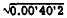is located right     Powers of 10 When the square root of 10, 1,000, 100,000, and so forth, is desired, the center index is used. That is, when the number of digits in a power of 10 is even, use the center index. The slide rule uses only the first three significant digits of a number. Thus, if the rule is used,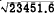must be considered as. Likewise, 1.43567 would be considered 1.43000, and so forth. For greater accuracy, other methods must be used. Practice problems. State which half of the A scale should be used for each of the following:Answers: 1. Left  2. Left  3. Left  4. Left  5. Right 6. Right 7. Left 8. Left Placing the Decimal Point To place the decimal point in the square root of a number, mark off the original number in groups of two as explained previously. For whole or mixed numbers, the number of groups marked off is the number of digits including end zeros to the left of the decimal point in the root. The following problems illustrate this: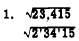Three digits to left of decimal point in square root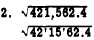Three digits to left of decimal point in square root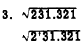Two digits to left of decimal point in square root For positive numbers less than one, there will be one zero in the square root between the decimal point and the first significant digit for every pair of zeros counted between the decimal point and the first significant digit of the original number. This is illustrated as follows:One zero before first digit in square rootTwo zeros before first digit in square rootNo zeros before first digit in square root EXAMPLE: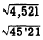(Two digits in left-hand group) Place the hairline over 452 on the right half of the A scale. Read the digit sequence of the root, 672, on the D scale under the hairline. Since there are two groups in the original number, there are two digits to the left of the decimal point in the root. Thus, EXAMPLE: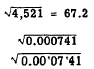(First figure is zero in this group) Place the hairline over 741 on the left half of the A scale. Read the digit sequence of the root, 272, under the hairline on the D scale. Since there is one pair of zeros to the left of the group containing the first digit, there is one zero between the decimal point and the first significant digit of the root. Thus,Practice problems. Evaluate each of the following by means of a slide rule: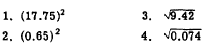Answers: 1. 315  2. 0.422  3. 3.07 4. 0.272